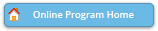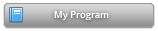#### Abstract Details

 Activity Number: 298 - Model/Variable Selection and Model Evaluation Type: Contributed Date/Time: Tuesday, July 30, 2019 : 8:30 AM to 10:20 AM Sponsor: Biometrics Section Abstract #304887 Title: Stochastic Covariates in Poisson Regression Author(s): Evrim Oral* Companies: LSUHSC School of Public Health, Department of Biostatistics Keywords: Count data; Stochastic covariates; Modified maximum likelihood; Order statistics; Outliers; Robustness Abstract: In finding the maximum likelihood estimators of a Poisson regression model, likelihood equations must be solved iteratively since they are intractable. Solving these equations by iterations can be problematic; for example the “poisson” command in Stata is known to encounter problems in locating the maximum. Oral (2017) derived explicit maximum likelihood-based estimators of Poisson regression model and studied their properties. In a traditional Poisson regression model, an important assumption is that the covariates are fixed by design. In real-life applications, however, they are most likely to be stochastic in nature. Oral (2002, 2006) derived modified maximum likelihood estimators in binary regression for the case when there is one stochastic covariate in the model. In this study, we extend the work of Oral (2006) to the models where the response variable is from count data. We show that when the covariate is stochastic, the new estimators are more precise than those obtained by the traditional Poisson regression using simulations. We illustrate our theoretical results using real life data from Emergency department visits.

Authors who are presenting talks have a * after their name.